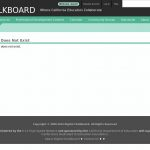# Tag Archives: equations and inequalities#### Linear Functions

September 24th, 2018

In this tutorial, “Linear functions of the form f(x) = a x + b and the properties of their graphs are explored interactively using an applet.” The applet allows students to manipulate variables to discover the changes in intercepts and Read more#### Solving Linear Inequalities: Introduction and Formatting

September 24th, 2018

From Elizabeth Stapel at Purplemath, this module helps students understand how to solve linear inequalities with an introduction to the topic using both inequality notation and a graphic representation to express the inequality.#### Linear Functions

September 24th, 2018

In this tutorial, “Linear functions of the form f(x) = a x + b and the properties of their graphs are explored interactively using an applet.” The applet allows students to manipulate variables to discover the changes in intercepts and Read more#### Solving Linear Inequalities: Introduction and Formatting

September 24th, 2018

From Elizabeth Stapel at Purplemath, this module helps students understand how to solve linear inequalities with an introduction to the topic using both inequality notation and a graphic representation to express the inequality.